## Example Questions

← Previous 1

### Example Question #1 : How To Find The Slope Of Perpendicular Lines

Two points on line m are (3,7) and (-2, 5).  Line k is perpendicular to line m.  What is the slope of line k?

2/5

-5/2

0

-5

3

-5/2

Explanation:

The slope of line m is the (y2 - y1) / (x- x1) = (5-7) / (-2 - 3)

= -2 / -5

= 2/5

To find the slope of a line perpendicular to a given line, we must take the negative reciprocal of the slope of the given line.

Thus the slope of line k is the negative reciprocal of 2/5 (slope of line m), which is -5/2.

### Example Question #2 : How To Find The Slope Of Perpendicular Lines

The equation of a line is: 8x + 16y = 48

What is the slope of a line that runs perpendicular to that line?

2

-2

-1/8

8

-1/4

2

Explanation:

First, solve for the equation of the line in the form of y = mx + b so that you can determine the slope, m of the line:

8x + 16y = 48

16y = -8x + 48

y = -(8/16)x + 48/16

y = -(1/2)x + 3

Therefore the slope (or m) = -1/2

The slope of a perpendicular line is the negative inverse of the slope.

m = - (-2/1) = 2

### Example Question #3 : How To Find The Slope Of Perpendicular Lines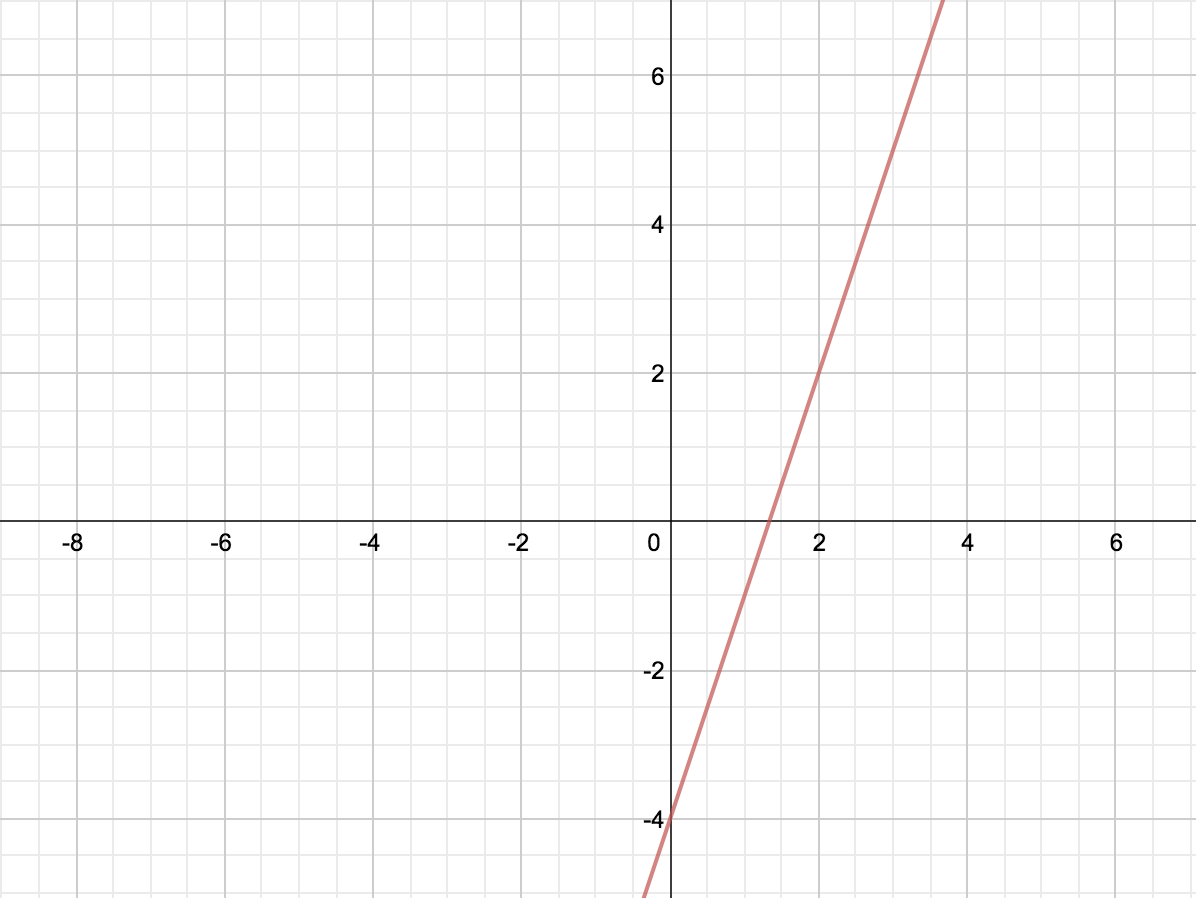What is the equation of a line perpendicular to the one above, passing through the point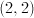?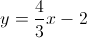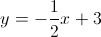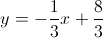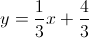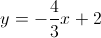Explanation:

Looking at the graph, we can tell the slope of the line is 3 with a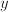-intercept of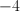, so the equation of the line is: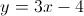A perpendicular line to this would have a slope of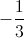, and would pass through the pointso it follows: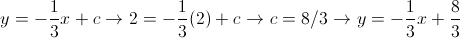### Example Question #4 : How To Find The Slope Of Perpendicular Lines

Line M passes through the points (2,2) and (3,–5).  Which of the following is perpendicular to line M?

y = 7x + 4

y = –7x – 5

y = (1/7)x + 3

y = –(1/7)x – 1

y = 7x – 6

y = (1/7)x + 3

Explanation:

First we find the slope of line M by using the slope formula (y– y1)/(x– x1).

(–5 – 2)/(3 – 2) = –7/1. This means the slope of Line M is –7.  A line perpendicular to Line M will have a negative reciprocal slope. Thus, the answer is = (1/7)x + 3.

### Example Question #1 : How To Find The Slope Of Perpendicular Lines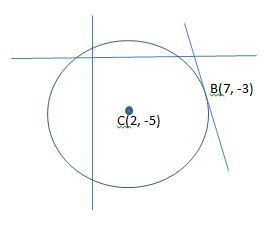Figure not drawn to scale.

In the figure above, a circle is centered at point C and a line is tangent to the circle at point B. What is the equation of the line?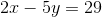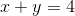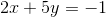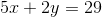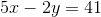Explanation:

We know that the line passes through point B, but we must calculate its slope in order to find the equation that defines the line. Because the line is tangent to the circle, it must make a right angle with the radius of the circle at point B. Therefore, the slope of the line is perpendicular to the slope of the radius that connects the center of the circle to point B. First, we can find the slope of the radius, and then we can determine the perpendicular slope.

The radius passes through points C and B. We can use the formula for the slope (represented as) between two points to find the slope of the radius.

Point C: (2,-5) and point B: (7,-3)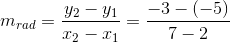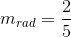This is the slope of the radius, but we need to find the slope of the line that is perpendicular to the radius. This value will be equal to the negative reciprocal.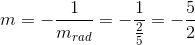Now we know the slope of the tangent line. We can use the point-slope formula to find the equation of the line. The formula is shown below.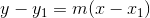Plug in the give point that lies on the tangent line (point B) and simplify the equation.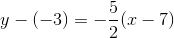Multiply both sides by two in order to remove the fraction.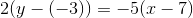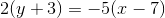Distribute both sides.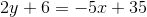Add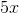to both sides.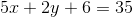Subtract six from both sides.The answer is.

### Example Question #1 : How To Find The Slope Of A Perpendicular Line

What is the slope of any line perpendicular to 2y = 4x +3 ?

2

½

– ½

– 4

– ½

Explanation:

First, we must solve the equation for y to determine the slope:  y = 2x + 3/2

By looking at the coefficient in front of x, we know that the slope of this line has a value of 2. To fine the slope of any line perpendicular to this one, we take the negative reciprocal of it:

slope = m , perpendicular slope = – 1/m

slope = 2 , perpendicular slope = – 1/2

### Example Question #1 : How To Find The Slope Of A Perpendicular Line

What line is perpendicular to 2x + y = 3 at (1,1)?

x – 2y = -1

3x + 2y = 1

2x + 3y = 1

x + 2y - 3

x – 2y = -1

Explanation:

Find the slope of the given line.  The perpendicular slope will be the opposite reciprocal of the original slope.  Use the slope-intercept form (y = mx + b) and substitute in the given point and the new slope to find the intercept, b.  Convert back to standard form of an equation:  ax + by =  c.

### Example Question #1 : How To Find The Slope Of A Perpendicular Line

What is the slope of the line perpendicular to the line given by the equation

6x – 9y +14 = 0

6

2/3

-1/6

-3/2

-2/3

-3/2

Explanation:

First rearrange the equation so that it is in slope-intercept form, resulting in y=2/3 x + 14/9. The slope of this line is 2/3, so the slope of the line perpendicular will have the opposite reciprocal as a slope, which is -3/2.

### Example Question #4 : How To Find The Slope Of A Perpendicular Line

What is the slope of the line perpendicular to the line represented by the equation y = -2x+3?

2

1/2

2/3

-2/3

-1/2

1/2

Explanation:

Perpendicular lines have slopes that are the opposite of the reciprocal of each other. In this case, the slope of the first line is -2. The reciprocal of -2 is -1/2, so the opposite of the reciprocal is therefore 1/2.

### Example Question #5 : How To Find The Slope Of A Perpendicular Line

Find the slope of a line perpendicular to the line y = –3x – 4.

–3

1/3

1/4

4